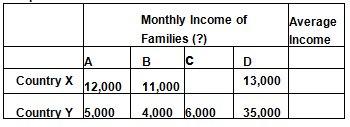# Assume that there are only four families each in two countries. Study the given table below carefully and answer the questions that follow:

Assume that there are only four families each in two countries. Study the given table below carefully and answer the questions that follow:(i) Fill in the blanks in a way that both country X and Y have the same average income.
(ii) Now say, which country is better off and why ?

(i) Country Y’s average income =
(5000 + 4000 + 6000 + 35000)/4 = 50,000
To maintain average income of country Y to be same as country X.
Family C’s monthly income in country X be 14,000 which is calculated as follows—If
(12,000+11,000 + Family C’s income + 13,000 )/4 = 12,500
( 36,000 + Family C’s income)/4 = 12,500
36,000+ Family C’s income = 50,000
Family C’s income = Rs. 14,000
(ii) Country X is better off than country Y inspite of the fact that both countries have the same average income because country X has equitable distribution of income among people in a country (i.e. people are neither very rich nor extremely poor). While most citizens in country Y are poor and one family D is extremely rich.